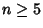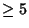## Solvable Group

A solvable group is a group whose composition indices are all Prime Numbers. Equivalently, a solvable group is a Group having a ``normal series'' such that each ``normal factor'' is Abelian. The term solvable derives from this type of group's relationship to Galois's Theorem, namely that the Symmetric Groupis insoluble forwhile it is solvable for, 2, 3, and 4. As a result, the Polynomial equations of degreeare not solvable using finite additions, multiplications, divisions, and root extractions.

Every Finite Group of order, every Abelian Group, and every Subgroup of a solvable group is solvable.